## Cube Root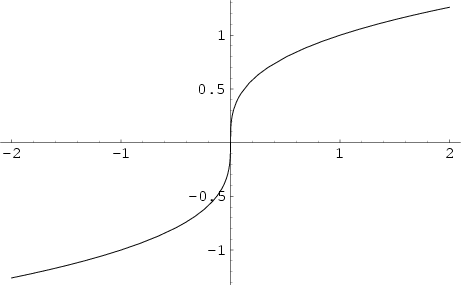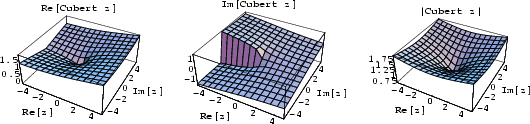Given a number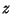, the cube root of, denoted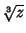or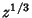(to the 1/3 Power), is a numbersuch that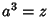. There are three (not necessarily distinct) cube roots for any number.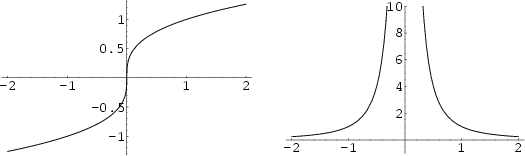For real arguments, the cube root is an Increasing Function, although the usual derivative test cannot be used to establish this fact at the Origin since the derivative approaches infinity there (as illustrated above).

See also Cube Duplication, Cubed, Delian Constant, Geometric Problems of Antiquity, k-Matrix, Square Root